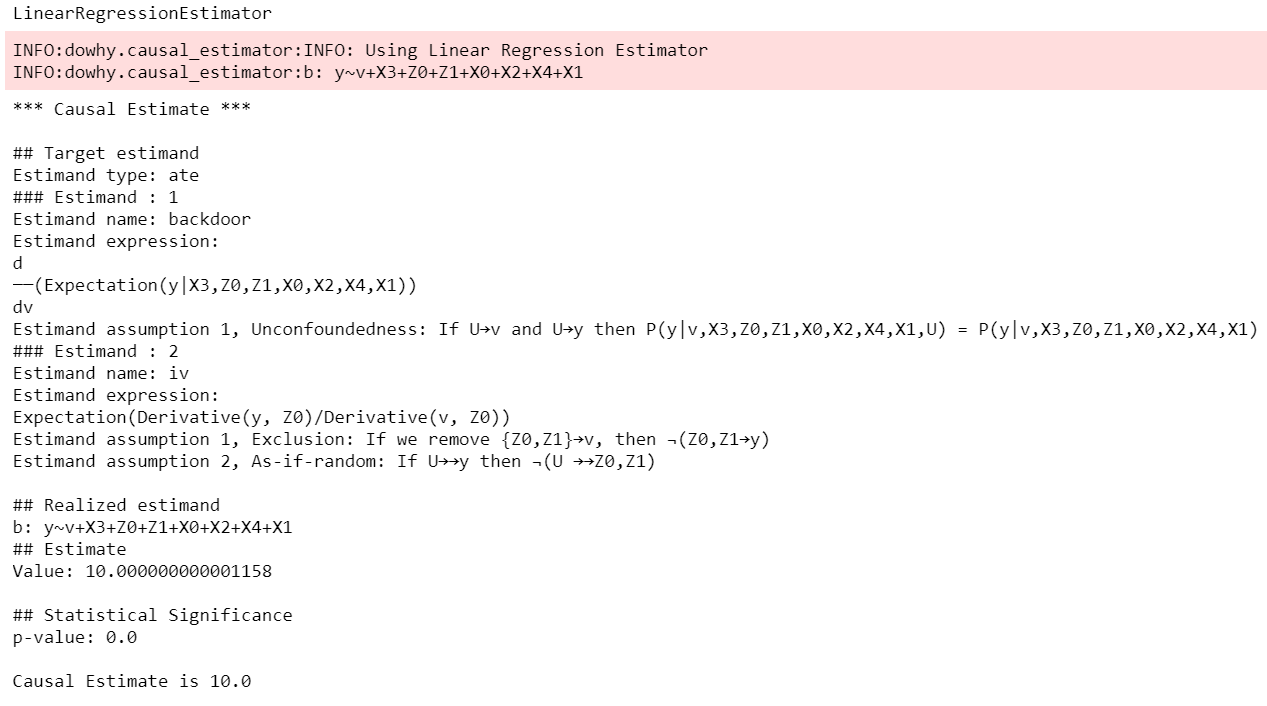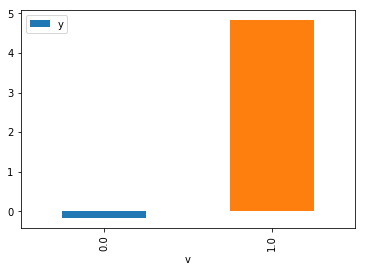# DoWhy | Making causal inference easy

Blog Post | Docs | Try it in a web browser!As computing systems are more frequently and more actively intervening in societally critical domains such as healthcare, education, and governance, it is critical to correctly predict and understand the causal effects of these interventions. Without an A/B test, conventional machine learning methods, built on pattern recognition and correlational analyses, are insufficient for causal reasoning.

Much like machine learning libraries have done for prediction, “DoWhy” is a Python library that aims to spark causal thinking and analysis. DoWhy provides a unified interface for causal inference methods and automatically tests many assumptions, thus making inference accessible to non-experts.

For a quick introduction to causal inference, check out amit-sharma/causal-inference-tutorial. We also gave a more comprehensive tutorial at the ACM Knowledge Discovery and Data Mining (KDD 2018) conference: causalinference.gitlab.io/kdd-tutorial.

Documentation for DoWhy is available at microsoft.github.io/dowhy.

## The need for causal inference

Predictive models uncover patterns that connect the inputs and outcome in observed data. To intervene, however, we need to estimate the effect of changing an input from its current value, for which no data exists. Such questions, involving estimating a counterfactual, are common in decision-making scenarios.

• Will it work?
• Does a proposed change to a system improve people’s outcomes?

• Why did it work?
• What led to a change in a system’s outcome?

• What should we do?
• What changes to a system are likely to improve outcomes for people?

• What are the overall effects?
• How does the system interact with human behavior?

• What is the effect of a system’s recommendations on people’s activity?

Answering these questions requires causal reasoning. While many methods exist for causal inference, it is hard to compare their assumptions and robustness of results. DoWhy makes three contributions,

1. Provides a principled way of modeling a given problem as a causal graph so that all assumptions explicit.

2. Provides a unified interface for many popular causal inference methods, combining the two major frameworks of graphical models and potential outcomes.

3. Automatically tests for the validity of assumptions if possible and assesses the robustness of the estimate to violations.

## Sample causal inference analysis in DoWhy

Most DoWhy analyses for causal inference take 4 lines to write, assuming a pandas dataframe df that contains the data:

```import dowhy
from dowhy.do_why import CausalModel
import dowhy.datasets

data = dowhy.datasets.linear_dataset(
beta=10,
num_common_causes=5,
num_instruments=2,
num_samples=10000,
treatment_is_binary=True)
```

DoWhy supports two formats for providing the causal graph: gml (preferred) and dot. After loading in the data, we use the four main operations in DoWhy: model, estimate, identify and refute:

```# Create a causal model from the data and given graph.
model = CausalModel(
data=data["df"],
treatment=data["treatment_name"],
outcome=data["outcome_name"],
graph=data["gml_graph"])

# Identify causal effect and return target estimands
identified_estimand = model.identify_effect()

# Estimate the target estimand using a statistical method.
estimate = model.estimate_effect(identified_estimand,
method_name="backdoor.propensity_score_matching")

# Refute the obtained estimate using multiple robustness checks.
refute_results = model.refute_estimate(identified_estimand, estimate,
method_name="random_common_cause")
```

DoWhy stresses on the interpretability of its output. At any point in the analysis, you can inspect the untested assumptions, identified estimands (if any) and the estimate (if any). Here’s a sample output of the linear regression estimator.For detailed code examples, check out the Jupyter notebooks in docs/source/, or try them online at Binder.

## A High-level Pandas API

We’ve made an even simpler API for dowhy which is a light layer on top of the standard one. The goal was to make causal analysis much more like regular exploratory analysis. To use this API, simply import `dowhy.api`. This will magically add the `causal` namespace to your `pandas.DataFrame` s. Then, you can use the namespace as follows.

```import dowhy.api
import dowhy.datasets

data = dowhy.datasets.linear_dataset(beta=5,
num_common_causes=1,
num_instruments = 0,
num_samples=1000,
treatment_is_binary=True)

# data['df'] is just a regular pandas.DataFrame
data['df'].causal.do(x='v',
variable_types={'v': 'b', 'y': 'c', 'X0': 'c'},
outcome='y',
common_causes=['X0']).groupby('v').mean().plot(y='y', kind='bar')
```The `do` method in the causal namespace generates a random sample from \$P(outcome|do(X=x))\$ of the same length as your data set, and returns this outcome as a new `DataFrame`. You can continue to perform the usual `DataFrame` operations with this sample, and so you can compute statistics and create plots for causal outcomes!

The `do` method is built on top of the lower-level `dowhy` objects, so can still take a graph and perform identification automatically when you provide a graph instead of `common_causes`.

## Installation

Requirements

DoWhy support Python 3+. It requires the following packages:

• numpy

• scipy

• scikit-learn

• pandas

• networkx (for analyzing causal graphs)

• matplotlib (for general plotting)

• sympy (for rendering symbolic expressions)

Install DoWhy and its dependencies by running this from the top-most folder of the repo.

```python setup.py install
```

If you face any problems, try installing dependencies manually.

```pip install -r requirements.txt
```

Optionally, if you wish to input graphs in the dot format, then install pydot (or pygraphviz).

For better-looking graphs, you can optionally install pygraphviz. To proceed, first install graphviz and then pygraphviz (on Ubuntu and Ubuntu WSL).

```sudo apt install graphviz libgraphviz-dev graphviz-dev pkg-config
## from https://github.com/pygraphviz/pygraphviz/issues/71
pip install pygraphviz --install-option="--include-path=/usr/include/graphviz" \
--install-option="--library-path=/usr/lib/graphviz/"
```

Keep in mind that pygraphviz installation can be problematic on the latest versions of Python3. Tested to work with Python 3.5.

## Graphical Models and Potential Outcomes: Best of both worlds

DoWhy builds on two of the most powerful frameworks for causal inference: graphical models and potential outcomes. It uses graph-based criteria and do-calculus for modeling assumptions and identifying a non-parametric causal effect. For estimation, it switches to methods based primarily on potential outcomes.

## A unifying language for causal inference

DoWhy is based on a simple unifying language for causal inference. Causal inference may seem tricky, but almost all methods follow four key steps:

1. Model a causal inference problem using assumptions.

2. Identify an expression for the causal effect under these assumptions (“causal estimand”).

3. Estimate the expression using statistical methods such as matching or instrumental variables.

4. Finally, verify the validity of the estimate using a variety of robustness checks.

This workflow can be captured by four key verbs in DoWhy:

• model

• identify

• estimate

• refute

Using these verbs, DoWhy implements a causal inference engine that can support a variety of methods. model encodes prior knowledge as a formal causal graph, identify uses graph-based methods to identify the causal effect, estimate uses statistical methods for estimating the identified estimand, and finally refute tries to refute the obtained estimate by testing robustness to assumptions.

DoWhy brings three key differences compared to available software for causal inference:

Explicit identifying assumptions

Assumptions are first-class citizens in DoWhy.

Each analysis starts with a building a causal model. The assumptions can be viewed graphically or in terms of conditional independence statements. Wherever possible, DoWhy can also automatically test for stated assumptions using observed data.

Separation between identification and estimation

Identification is the causal problem. Estimation is simply a statistical problem.

DoWhy respects this boundary and treats them separately. This focuses the causal inference effort on identification, and frees up estimation using any available statistical estimator for a target estimand. In addition, multiple estimation methods can be used for a single identified_estimand and vice-versa.

Automated robustness checks

What happens when key identifying assumptions may not be satisfied?

The most critical, and often skipped, part of causal analysis is checking the robustness of an estimate to unverified assumptions. DoWhy makes it easy to automatically run sensitivity and robustness checks on the obtained estimate.

Finally, DoWhy is easily extensible, allowing other implementations of the four verbs to co-exist (we hope to integrate with external implementations in the future). The four verbs are mutually independent, so their implementations can be combined in any way.

Below are more details about the current implementation of each of these verbs.

## Model a causal problem

DoWhy creates an underlying causal graphical model for each problem. This serves to make each causal assumption explicit. This graph need not be complete—you can provide a partial graph, representing prior knowledge about some of the variables. DoWhy automatically considers the rest of the variables as potential confounders.

Currently, DoWhy supports two formats for graph input: gml (preferred) and dot. We strongly suggest to use gml as the input format, as it works well with networkx. You can provide the graph either as a .gml file or as a string. If you prefer to use dot format, you will need to install additional packages (pydot or pygraphviz, see the installation section above). Both .dot files and string format are supported.

While not recommended, you can also specify common causes and/or instruments directly instead of providing a graph.

## Identify a target estimand under the model

Based on the causal graph, DoWhy finds all possible ways of identifying a desired causal effect based on the graphical model. It uses graph-based criteria and do-calculus to find potential ways find expressions that can identify the causal effect.

## Estimate causal effect based on the identified estimand

DoWhy supports methods based on both back-door criterion and instrumental variables. It also provides a non-parametric permutation test for testing the statistical significance of obtained estimate.

Currently supported back-door criterion methods.

• Methods based on estimating the treatment assignment
• Propensity-based Stratification

• Propensity Score Matching

• Inverse Propensity Weighting

• Methods based on estimating the response surface
• Regression

Currently supported methods based on instrumental variables.

• Binary Instrument/Wald Estimator

• Regression discontinuity

## Refute the obtained estimate

Having access to multiple refutation methods to verify a causal inference is a key benefit of using DoWhy.

DoWhy supports the following refutation methods.

• Placebo Treatment

• Subset validation